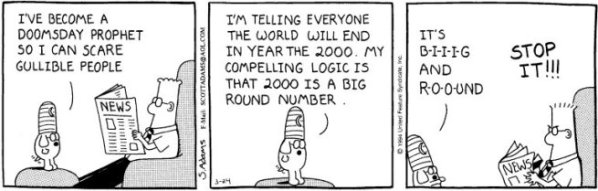# Rounding numbers

Rounding numbers is a very simple technique that trades accuracy for ease of calculation. It enables the creation of satisfactory approximations that are easier to work with. The numbers are rounded to a number with the desired number of decimal places that is closest to the starting number. Numbers can be rounded up or rounded down to the nearest value with fewer decimal places.
Example: Let us say we want to round a number with one decimal place to an integer. If the last digit behind the decimal point is 5 or greater than 5, then we round up. If the last digit is lesser than 5, we round down. So, if we have the value 12.6 and we want to round it to the nearest integer, 12.6 will be rounded to the value of 13. If the value we need rounded is, for example 17.2, then we round it down to the value of 17.## Rounding numbers exams for teachers

 Exam Name File Size Downloads Upload date Rounding numbers – very easy 144.1 kB 2716 September 3, 2019 Rounding numbers – easy 145.2 kB 2811 September 3, 2019 Rounding numbers – medium 146.8 kB 3140 September 3, 2019 Rounding numbers – hard 151.9 kB 2464 September 3, 2019 Rounding numbers – very hard 151.5 kB 2589 September 3, 2019